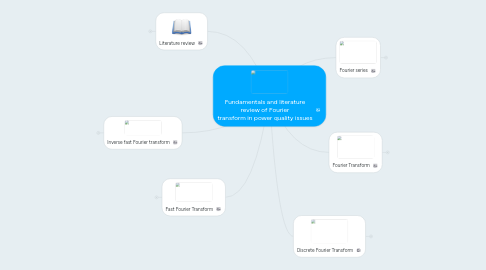# Fundamentals and literature review of Fourier transform in power quality issues

Jetzt loslegen. Gratis!Fundamentals and literature review of Fourier transform in power quality issues## 1. Fourier series

### 1.1. It is named after Jean Baptiste Joseph Fourier

1.1.1. The Fourier series is described in theory and problems of advanced calculus

## 2. Fourier Transform

### 2.1. It is used to transform signals between

2.1.1. The domain of time

2.1.2. The frecuence domain

## 4. Fast Fourier Transform

### 4.1. It is an efficient algorithm to compute the discrete Fourier transform and its inverse

4.1.1. There are two algorithms

4.1.1.1. Decimation in time

4.1.1.2. Decimation in frequency